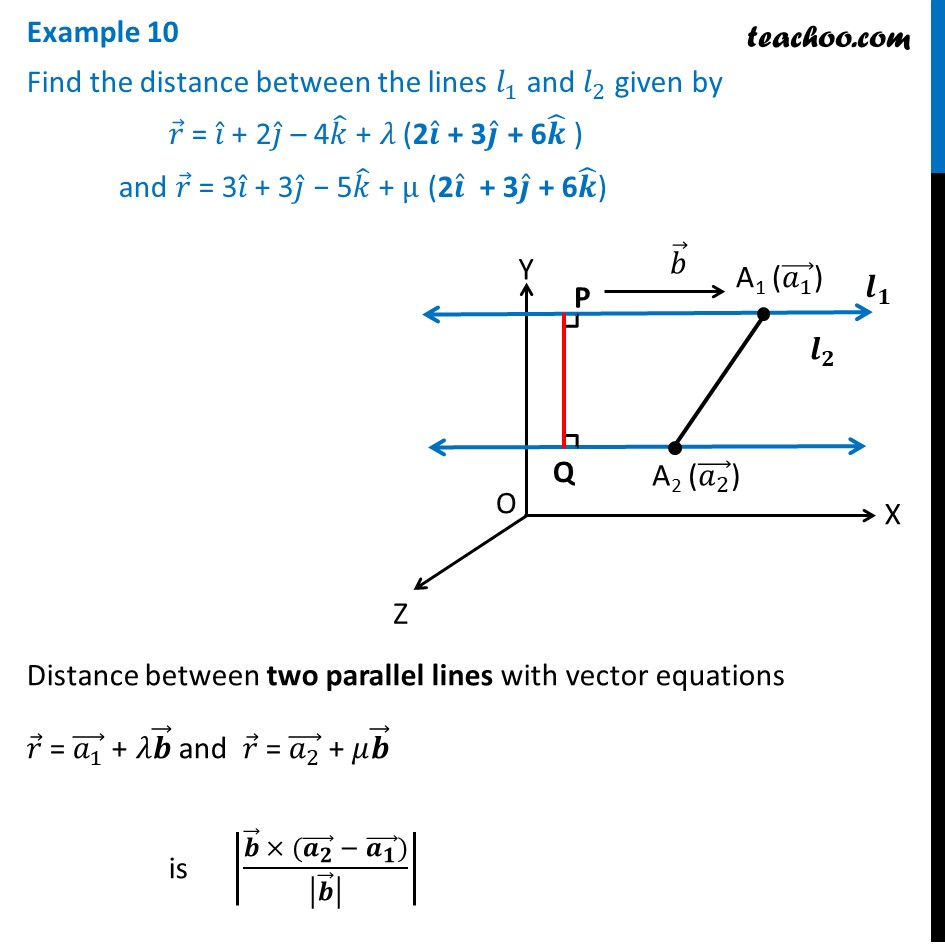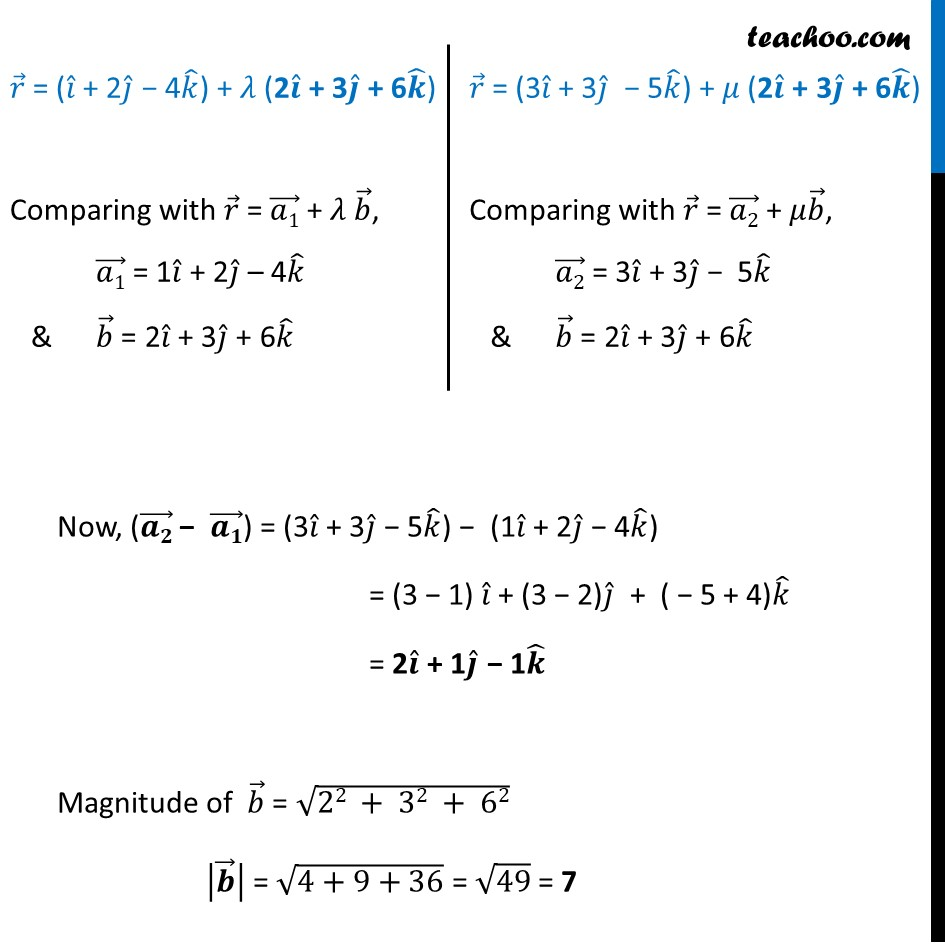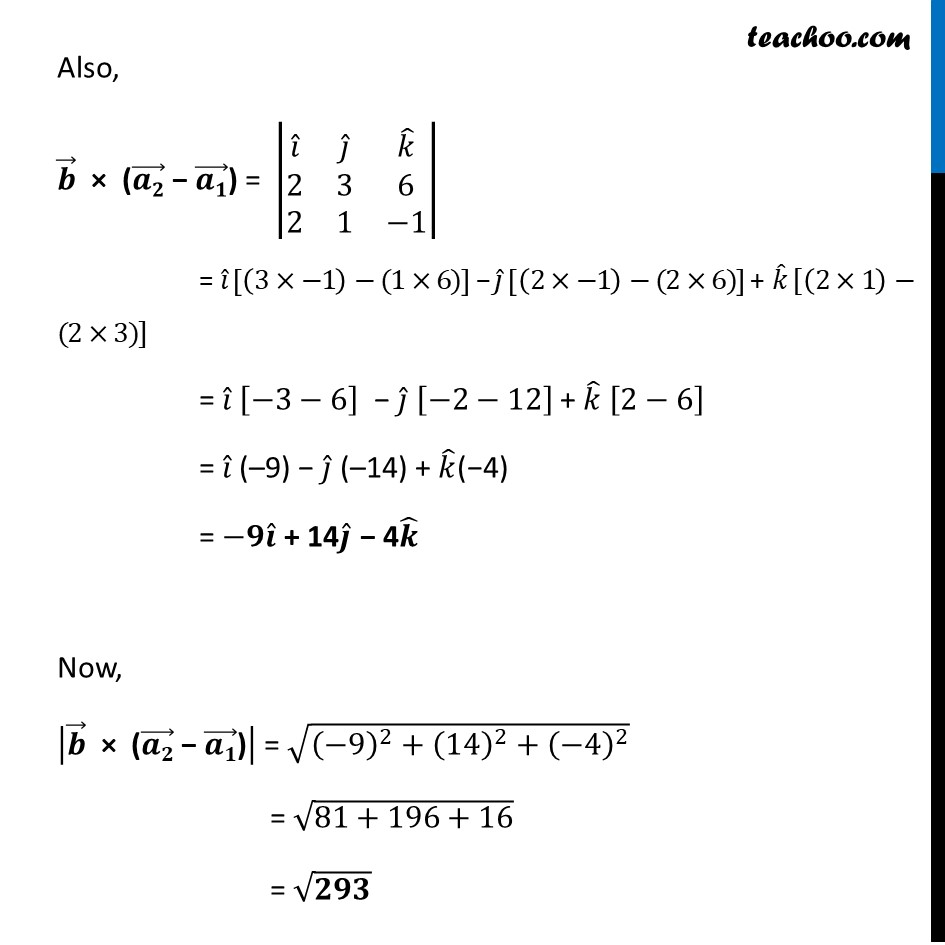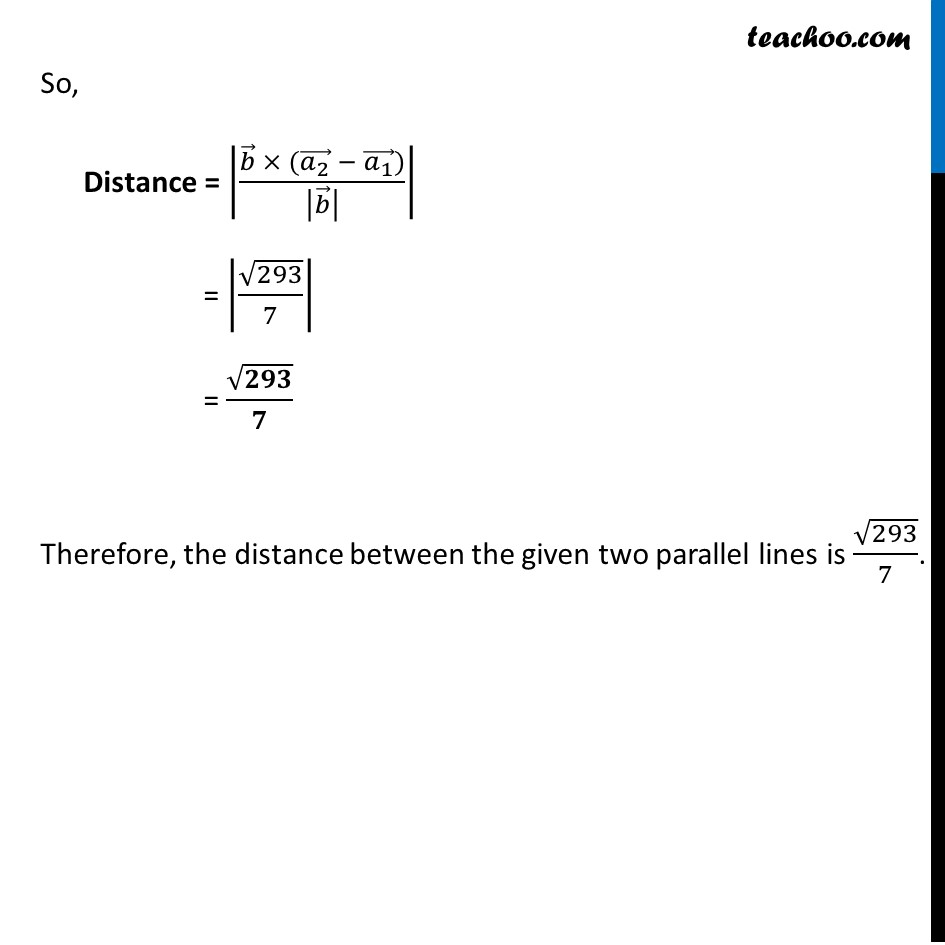Examples

Chapter 11 Class 12 Three Dimensional Geometry
Serial order wiseLearn in your speed, with individual attention - Teachoo Maths 1-on-1 Class

### Transcript

Example 10 Find the distance between the lines 𝑙_1 and 𝑙_2 given by 𝑟 ⃗ = 𝑖 ̂ + 2𝑗 ̂ – 4𝑘 ̂ + 𝜆 (2𝒊 ̂ + 3𝒋 ̂ + 6𝒌 ̂ ) and 𝑟 ⃗ = 3𝑖 ̂ + 3𝑗 ̂ − 5𝑘 ̂ + μ (2𝒊 ̂ + 3𝒋 ̂ + 6𝒌 ̂)Distance between two parallel lines with vector equations 𝑟 ⃗ = (𝑎_1 ) ⃗ + 𝜆𝒃 ⃗ and 𝑟 ⃗ = (𝑎_2 ) ⃗ + 𝜇𝒃 ⃗ is |(𝒃 ⃗ × ((𝒂_𝟐 ) ⃗ − (𝒂_𝟏 ) ⃗))/|𝒃 ⃗ | | 𝑟 ⃗ = (𝑖 ̂ + 2𝑗 ̂ − 4𝑘 ̂) + 𝜆 (2𝒊 ̂ + 3𝒋 ̂ + 6𝒌 ̂) Comparing with 𝑟 ⃗ = (𝑎1) ⃗ + 𝜆 𝑏 ⃗, (𝑎1) ⃗ = 1𝑖 ̂ + 2𝑗 ̂ – 4𝑘 ̂ & 𝑏 ⃗ = 2𝑖 ̂ + 3𝑗 ̂ + 6𝑘 ̂ 𝑟 ⃗ = (3𝑖 ̂ + 3𝑗 ̂ − 5𝑘 ̂) + 𝜇 (2𝒊 ̂ + 3𝒋 ̂ + 6𝒌 ̂) Comparing with 𝑟 ⃗ = (𝑎2) ⃗ + 𝜇𝑏 ⃗, (𝑎2) ⃗ = 3𝑖 ̂ + 3𝑗 ̂ − 5𝑘 ̂ & 𝑏 ⃗ = 2𝑖 ̂ + 3𝑗 ̂ + 6𝑘 ̂ Now, ((𝒂𝟐) ⃗ − (𝒂𝟏) ⃗) = (3𝑖 ̂ + 3𝑗 ̂ − 5𝑘 ̂) − (1𝑖 ̂ + 2𝑗 ̂ − 4𝑘 ̂) = (3 − 1) 𝑖 ̂ + (3 − 2)𝑗 ̂ + ( − 5 + 4)𝑘 ̂ = 2𝒊 ̂ + 1𝒋 ̂ − 1𝒌 ̂ Magnitude of 𝑏 ⃗ = √(22 + 32 + 62) |𝒃 ⃗ | = √(4+9+36) = √49 = 7 Also, 𝒃 ⃗ × ((𝒂𝟐) ⃗ − (𝒂𝟏) ⃗) = |■8(𝑖 ̂&𝑗 ̂&𝑘 ̂@2&3&6@2&1&−1)| = 𝑖 ̂ [(3×−1)−(1×6)] − 𝑗 ̂ [(2×−1)−(2×6)] + 𝑘 ̂ [(2×1)−(2×3)] = 𝑖 ̂ [−3−6] − 𝑗 ̂ [−2−12] + 𝑘 ̂ [2−6] = 𝑖 ̂ (–9) − 𝑗 ̂ (–14) + 𝑘 ̂(−4) = −𝟗𝒊 ̂ + 14𝒋 ̂ − 4𝒌 ̂ Now, |𝒃 ⃗" × (" (𝒂𝟐) ⃗" − " (𝒂𝟏) ⃗")" | = √((−9)^2+(14)^2+(−4)^2 ) = √(81+196+16) = √𝟐𝟗𝟑 So, Distance = |(𝑏 ⃗ × ((𝑎_2 ) ⃗ − (𝑎_1 ) ⃗))/|𝑏 ⃗ | | = |√293/7| = √𝟐𝟗𝟑/𝟕 Therefore, the distance between the given two parallel lines is √293/7.